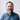# From One End to the Other in Ruby

A while back I tackled the following programming challenge:

From One End to the Other: Find the smallest possible (positive) integer that ends in a six such that if that six is removed and placed in front of the remaining digits of the number, the resulting number will be four times as large as the original.

My solution is below, and here:

https://gist.github.com/jwworth/5a5b3647a44b9a782896

I like that this solution is relatively fast, at 0.36 seconds. I like that it doesn’t imply what the answer might be by having a ceiling to the search— the code will run forever if my conditional is wrong. I like that it uses the `rotate` method, something I didn’t know existed until I tried to solve this problem.

I don’t like that this code isn’t tested, and that it turns the integer into a string. String manipulation is one of the best features of Ruby, but I rely on it too often.

This code came after a major refactor, viewable in the Gist history. My first draft arrived at the same solution, but telegraphed the answer via a range, which is a little misleading. Ironically, this is closer to the type of code I would have written when I was just getting started as a programmer. I didn’t know how to find the answer first then, so I had to write code that made zero assumptions.

``````# from_one_end_to_the_other.rb

i = 6
loop do
digits = i.to_s.split('')
if digits.last == '6' && digits.rotate(-1).join.to_i == (i * 4)
puts i
break
end
i = i + 2
end

# 153846``````

For the curious, I also solved this in Elm. No syntax highlighting available at the time of posting, so enjoy the bleeding edge:

``````import Graphics.Element exposing (show)
import Array exposing (..)
import String exposing (..)

magicNumber : Array Int
magicNumber =
let
isNumber number =
String.right 1 (toString(number)) == "6" &&
(String.append "6" (String.dropRight 1 (toString(number))))
== toString(number * 4)

in
Array.filter isNumber (initialize 100000 (\n -> n * 2))

main = show magicNumber
-- Array.fromList ``````

https://gist.github.com/jwworth/9315df793666708bfe8c

And finally, here is my solution in Lua:

``````function swap_first_and_last(t, n)
table.insert(t, 1, n)
end

for i = 6, 1000000, 2 do
local digits = {}
local count = 1

for x in string.gmatch(tostring(i), "%S") do
table.insert(digits, count, x)
count = count + 1
end

local last = table.remove(digits)

if last == "6" and swap_first_and_last(digits, last) == i * 4 then
print(i)
os.exit()
end
end

-- 153846``````

https://gist.github.com/jwworth/b7926816974edb2f2354

I’m still a Rubyist! Thanks to Josh Branchaud for posting this challenge.